# Grade 2 math 11.10b money problem solving list (dollars and cents)

##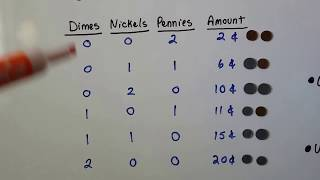By Joann's School

Solving a two-step word problem that involves money.# Solving problems with bar graphs 1

##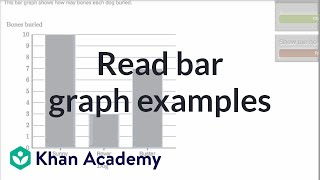By Khan Academy

Solving problems with bar graphs 1# Solving problems with bar graphs 2

##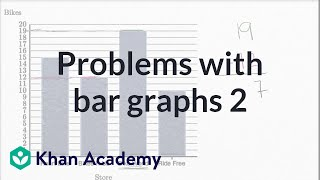By Khan Academy

Solving problems with bar graphs 2# Division Problem Solving

##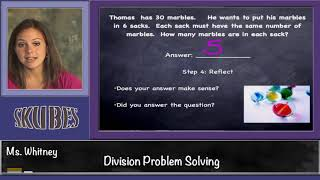By Skubes ed

Use multiplication and division within 100 to solve word problems in situations involving equal groups, arrays, and measurement quantities, e.g., by using drawings and equations with a symbol for the unknown number to represent the problem.1# Solving problems with pictographs 2

##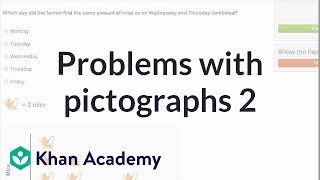By Khan Academy

Use picture graphs to solve word problems.# Solving a Geometry Word Problem by Using Quadratic Equations - Example 1

##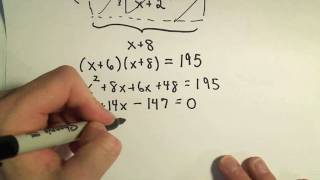By PatrickJMT

Solving a Geometry Word Problem by Using Quadratic Equations - Example 1# solve word problems using addition and subtraction of fractions

##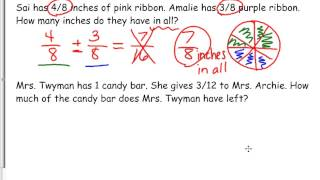By Kathleen Twyman

how to solve a word problem using addition and subtraction of fractions# Solving proportion word problems

##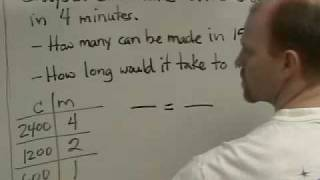By Duane Habecker

This is a video about solving proportion word problems.# Solving Math Word Problems

##By expertvillage

In math word problems it's important to figure out what the facts are and what is being asked for. Solve math word problems with tips from a math teacher in this video on solving math problems.# Solving Math Word Problems

##By expertvillage

In math word problems it's important to figure out what the facts are and what is being asked for. Solve math word problems with tips from a math teacher in this video on solving math problems.# How to use Ratios and Proportions to Solve Real World Problems

##By mrtedmartin

Instructor uses a Power Point presentation to demonstrate how to use ratios and proportions to solve problems real world problems. Unit rates are discussed and definitions are given. Solving unit rate problems are modeled and a calculator is used for computation.# Ex 2: Set Problem Solving - Find the Number of Element in the Union of Two Sets Using a Formula

##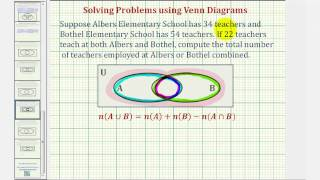By Mathispower4u

Ex 2: Set Problem Solving - Find the Number of Element in the Union of Two Sets Using a Formula# Constructing linear equations to solve word problems

##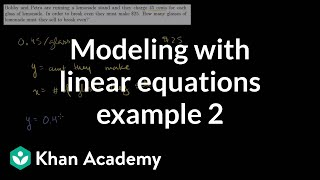By Khan Academy

Khan Academy presents Word Problem Solving 4 an educational video resource on math.# Solving Word Problems Involving Inequalities - Example 1

##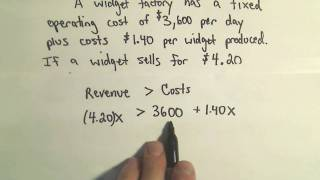By PatrickJMT

Youtube Presents Solving Word Problems Involving Inequalities - Example 1 an educational video resources on english language arts.# Solve Problems Involving Rates and Ratios

##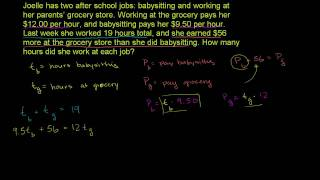By Khan Academy

Watch this video as the instructor clearly explains how to solve a word problem involving finding unit rates. When rates are expressed as a quantity of 1, such as 2 feet per second or 5 miles per hour, they are called unit rates. See how you can apply this process to help you solve similar problems.# Solve Problems Involving Rates and Ratios

##By Khan Academy

Watch this video as the instructor clearly explains how to solve a word problem involving finding unit rates. When rates are expressed as a quantity of 1 such as 2 feet per second or 5 miles per hour they are called unit rates. See how you can apply this process to help you solve similar problems.# How to Solve Similar Triangles Applications: Flagpole Problem

##By Math Class with Terry V

YouTube presents How to Solve Similar Triangles Applications: Flagpole Problem, an educational video resource on math.# Solving 2-Step Word Problems | Good To Know | WSKG

##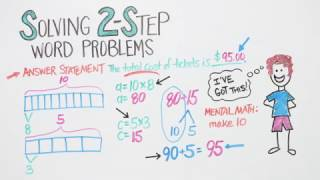By WSKG Public Media

solve two-step word problems using addition, subtraction, multiplication, and division.# Using Venn Diagrams to Solve Word Problems

##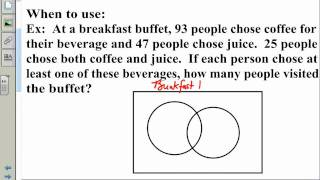By TeacherTube Math

The instructor shows how and when to use Venn Diagrams to solve word problems. He uses an interactive whiteboard to demonstrate the word problem that can be solved with Venn Diagrams. Urges students to label their charts and graphs. Teacher teaches clearly and provides good models.# Solving Problems Proving Geometric Relationships using Congruence and Similarity Criteria

##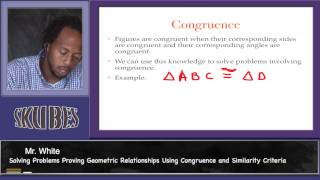By Skubes Ed

Use congruence and similarity criteria for triangles to solve problems and to prove relationships in geometric figures.